Скачать презентацию Caps and Swaps Floating rate securities Coupon

f01c82c04590199dd029cf0ede28f8f3.ppt

• Количество слайдов: 15Caps and Swaps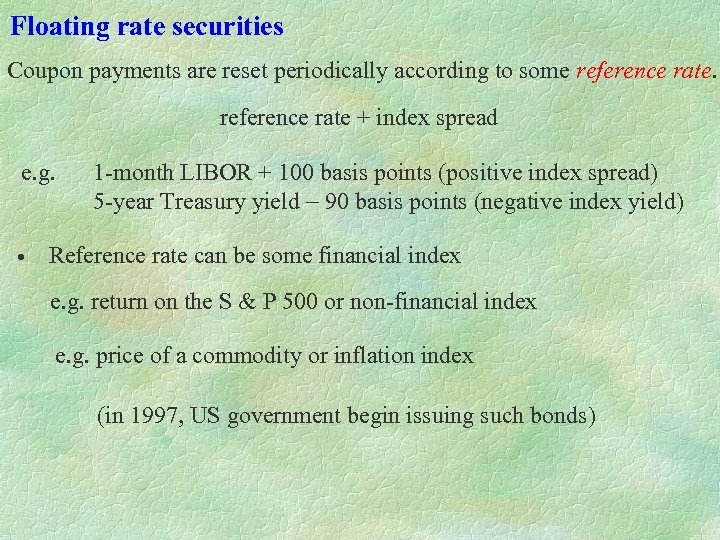Floating rate securities Coupon payments are reset periodically according to some reference rate + index spread e. g. 1 -month LIBOR + 100 basis points (positive index spread) 5 -year Treasury yield - 90 basis points (negative index yield) • Reference rate can be some financial index e. g. return on the S & P 500 or non-financial index e. g. price of a commodity or inflation index (in 1997, US government begin issuing such bonds)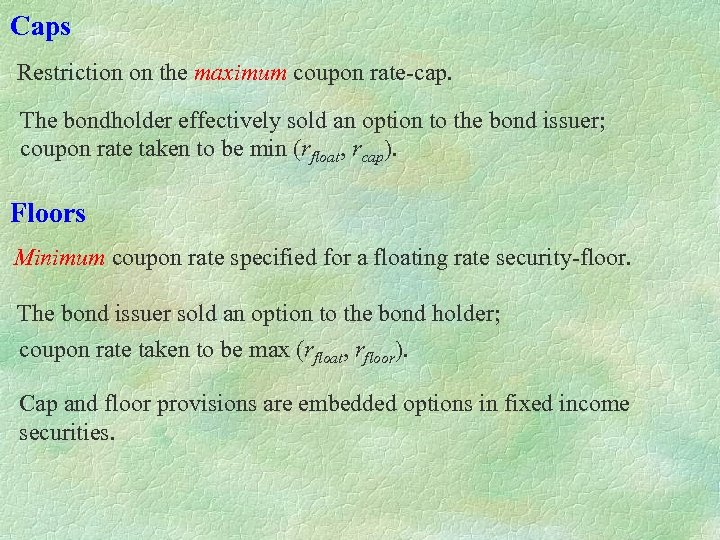Caps Restriction on the maximum coupon rate-cap. The bondholder effectively sold an option to the bond issuer; coupon rate taken to be min (rfloat, rcap). Floors Minimum coupon rate specified for a floating rate security-floor. The bond issuer sold an option to the bond holder; coupon rate taken to be max (rfloat, rfloor). Cap and floor provisions are embedded options in fixed income securities.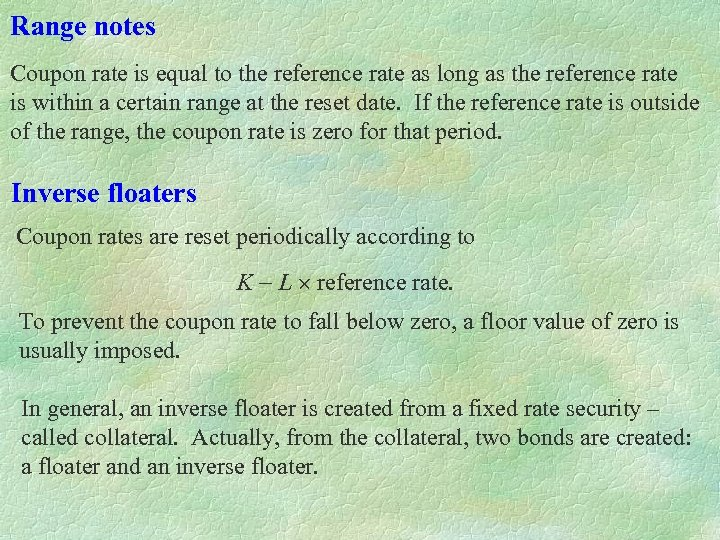Range notes Coupon rate is equal to the reference rate as long as the reference rate is within a certain range at the reset date. If the reference rate is outside of the range, the coupon rate is zero for that period. Inverse floaters Coupon rates are reset periodically according to K - L reference rate. To prevent the coupon rate to fall below zero, a floor value of zero is usually imposed. In general, an inverse floater is created from a fixed rate security – called collateral. Actually, from the collateral, two bonds are created: a floater and an inverse floater.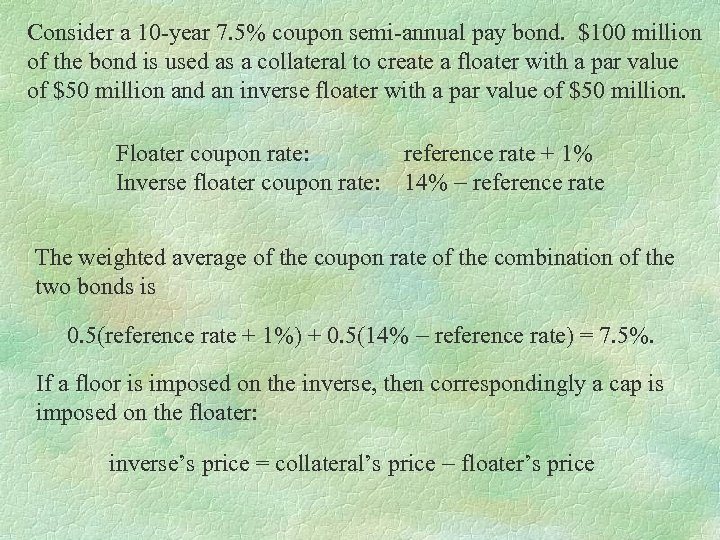Consider a 10 -year 7. 5% coupon semi-annual pay bond. \$100 million of the bond is used as a collateral to create a floater with a par value of \$50 million and an inverse floater with a par value of \$50 million. Floater coupon rate: reference rate + 1% Inverse floater coupon rate: 14% - reference rate The weighted average of the coupon rate of the combination of the two bonds is 0. 5(reference rate + 1%) + 0. 5(14% - reference rate) = 7. 5%. If a floor is imposed on the inverse, then correspondingly a cap is imposed on the floater: inverse’s price = collateral’s price - floater’s price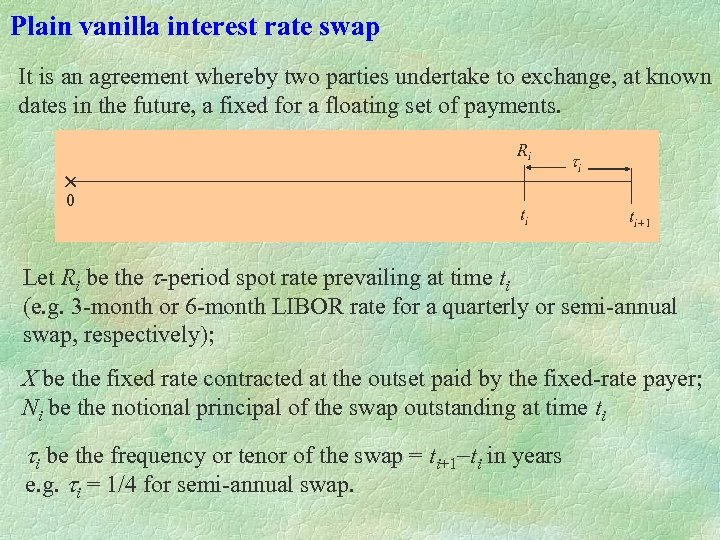Plain vanilla interest rate swap It is an agreement whereby two parties undertake to exchange, at known dates in the future, a fixed for a floating set of payments. Ri 0 ti ti ti+1 Let Ri be the t-period spot rate prevailing at time ti (e. g. 3 -month or 6 -month LIBOR rate for a quarterly or semi-annual swap, respectively); X be the fixed rate contracted at the outset paid by the fixed-rate payer; Ni be the notional principal of the swap outstanding at time ti ti be the frequency or tenor of the swap = ti+1 -ti in years e. g. ti = 1/4 for semi-annual swap.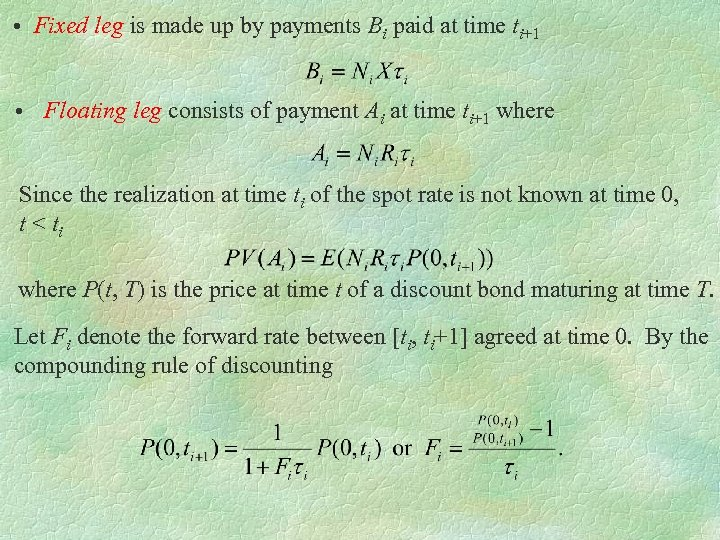• Fixed leg is made up by payments Bi paid at time ti+1 • Floating leg consists of payment Ai at time ti+1 where Since the realization at time ti of the spot rate is not known at time 0, t < ti where P(t, T) is the price at time t of a discount bond maturing at time T. Let Fi denote the forward rate between [ti, ti+1] agreed at time 0. By the compounding rule of discounting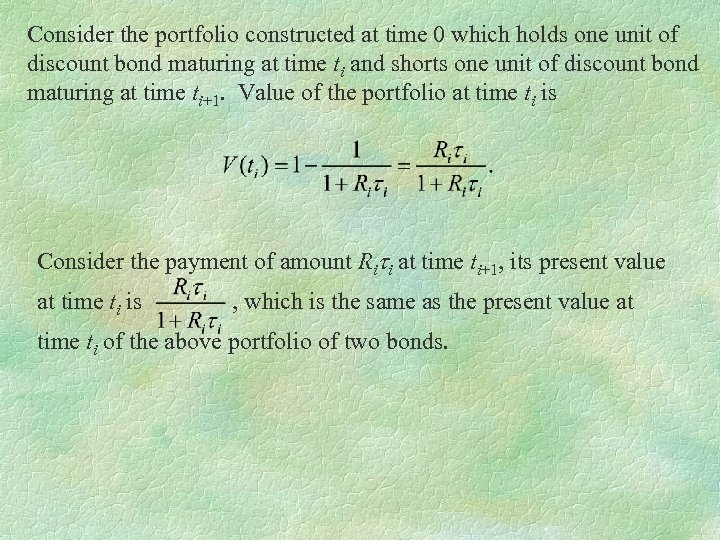Consider the portfolio constructed at time 0 which holds one unit of discount bond maturing at time ti and shorts one unit of discount bond maturing at time ti+1. Value of the portfolio at time ti is Consider the payment of amount Riti at time ti+1, its present value at time ti is , which is the same as the present value at time ti of the above portfolio of two bonds.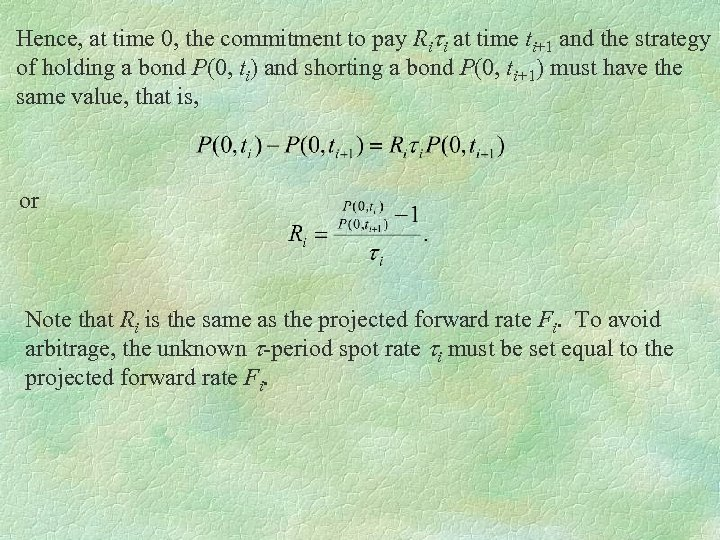Hence, at time 0, the commitment to pay Riti at time ti+1 and the strategy of holding a bond P(0, ti) and shorting a bond P(0, ti+1) must have the same value, that is, or Note that Ri is the same as the projected forward rate Fi. To avoid arbitrage, the unknown t-period spot rate ti must be set equal to the projected forward rate Fi.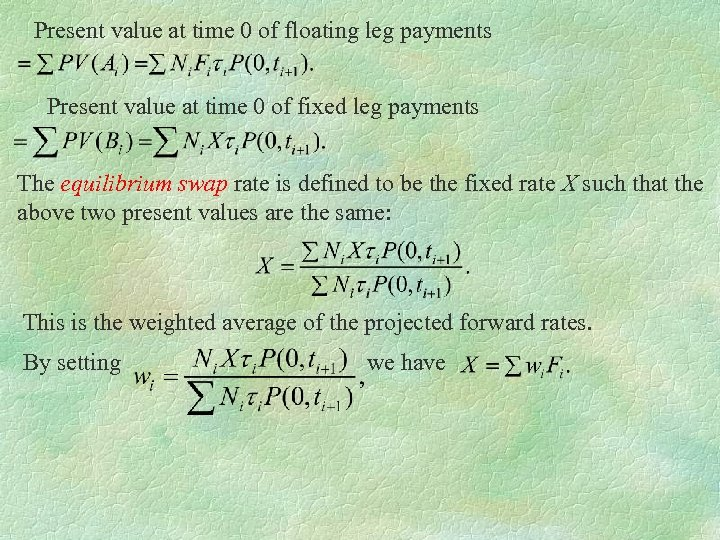Present value at time 0 of floating leg payments Present value at time 0 of fixed leg payments The equilibrium swap rate is defined to be the fixed rate X such that the above two present values are the same: This is the weighted average of the projected forward rates. By setting we have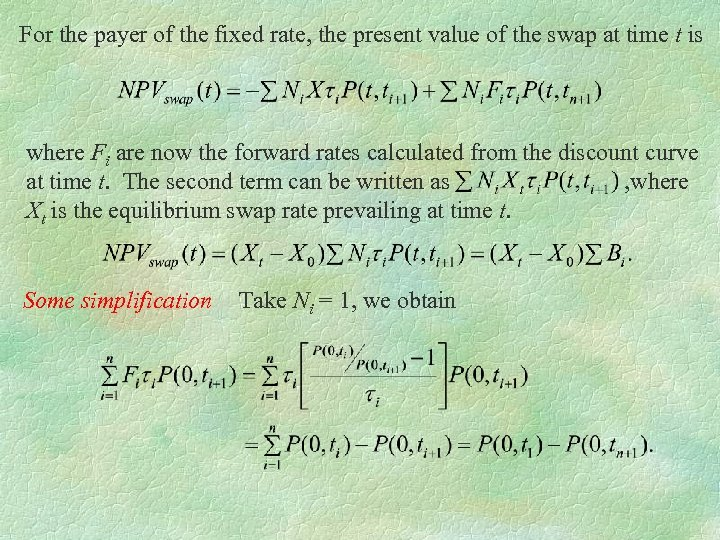For the payer of the fixed rate, the present value of the swap at time t is where Fi are now the forward rates calculated from the discount curve at time t. The second term can be written as , where Xt is the equilibrium swap rate prevailing at time t. Some simplification Take Ni = 1, we obtain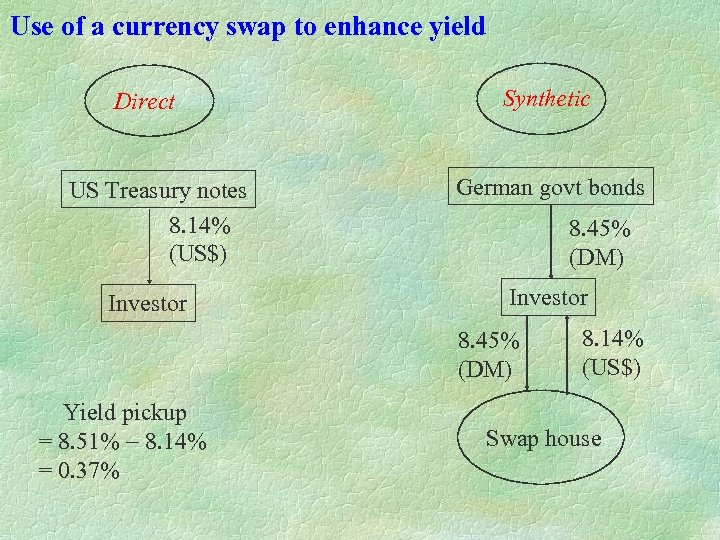Use of a currency swap to enhance yield Direct US Treasury notes 8. 14% (US\$) Investor Synthetic German govt bonds 8. 45% (DM) Investor 8. 45% (DM) Yield pickup = 8. 51% - 8. 14% = 0. 37% 8. 14% (US\$) Swap house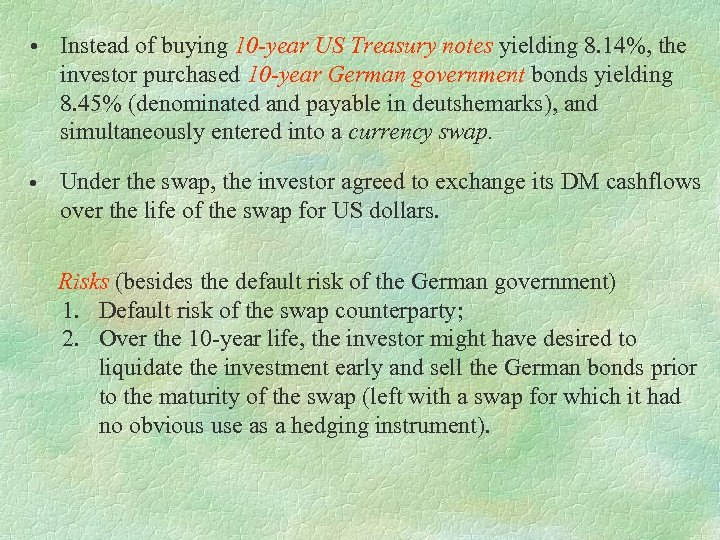• Instead of buying 10 -year US Treasury notes yielding 8. 14%, the investor purchased 10 -year German government bonds yielding 8. 45% (denominated and payable in deutshemarks), and simultaneously entered into a currency swap. • Under the swap, the investor agreed to exchange its DM cashflows over the life of the swap for US dollars. Risks (besides the default risk of the German government) 1. Default risk of the swap counterparty; 2. Over the 10 -year life, the investor might have desired to liquidate the investment early and sell the German bonds prior to the maturity of the swap (left with a swap for which it had no obvious use as a hedging instrument).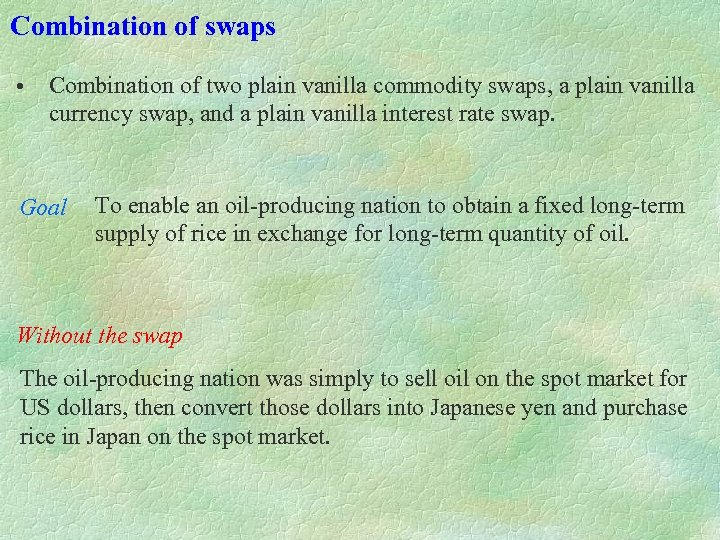Combination of swaps • Combination of two plain vanilla commodity swaps, a plain vanilla currency swap, and a plain vanilla interest rate swap. Goal To enable an oil-producing nation to obtain a fixed long-term supply of rice in exchange for long-term quantity of oil. Without the swap The oil-producing nation was simply to sell oil on the spot market for US dollars, then convert those dollars into Japanese yen and purchase rice in Japan on the spot market.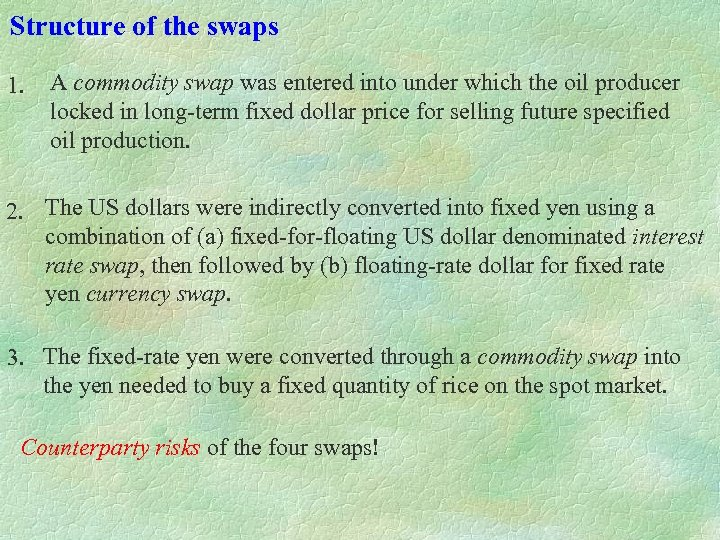Structure of the swaps 1. A commodity swap was entered into under which the oil producer locked in long-term fixed dollar price for selling future specified oil production. 2. The US dollars were indirectly converted into fixed yen using a combination of (a) fixed-for-floating US dollar denominated interest rate swap, then followed by (b) floating-rate dollar for fixed rate yen currency swap. 3. The fixed-rate yen were converted through a commodity swap into the yen needed to buy a fixed quantity of rice on the spot market. Counterparty risks of the four swaps!